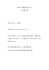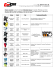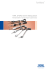# Optimal Design of Three- Link Planar Manipulators

## Transcription

Optimal Design of Three- Link Planar Manipulators
```70
Chapter 3
Optimal Design of ThreeLink Planar Manipulators
Using Grashof’s Criterion
Sarosh H. Patel
RISC Laboratory, University of Bridgeport, USA
Tarek Sobh
RISC Laboratory, University of Bridgeport, USA
ABSTRACT
The design of robotic manipulators is dictated by a set of pre-determined task descriptions and performance parameters. These performance parameters are often defined in terms of workspace dexterity,
manipulability, and accuracy. Many serial manipulator applications require that the manipulator have
full dexterity about a work piece or a pre-defined trajectory, that is, to approach the given point within
the workspace with all possible orientations about that point. Grashof’s criterion defines the mobility
of four-link closed chain mechanisms in relation to its link lengths. A simple assumption can convert
a three-link serial manipulator into a four-link closed chain so that its mobility can be studied using
Grashof’s criterion. With the help of Grashof’s criterion, it is possible not only to predict and simulate
the mobility of a manipulator during its design, but also to map and identify the fully-dexterous regions
within its workspace. Mapping of the dexterous workspace is helpful in efficient task placement and path
planning. Next, the authors propose a simple algorithm using Grashof’s criterion for determining the
optimal link lengths of a three-link manipulator, in order to achieve full dexterity at the desired regions
of the workspace. Finally, the authors test the generated design by applying joint angle limitations.
NOMENCLATURE
D: DMean: Dexterity index of the manipulator at a
point.
Mean dexterity index over a region or
trajectory.
DOI: 10.4018/978-1-4666-0176-5.ch003
N: Number of points along the trajectory.
dx,dy,dz: Dexterity indices about the X, Y and Z
axis.
α, β, γ: Yaw, pitch and roll angels of the endeffector.
a,b,c,d: Link lengths of the four-bar kinematic
chain.
Copyright © 2012, IGI Global. Copying or distributing in print or electronic forms without written permission of IGI Global is prohibited.
Optimal Design of Three-Link Planar Manipulators Using Grashof’s Criterion
manipulator.
θ1, θ2, θ3: Joint angles of the three-link planar
manipulator.
d: Distance between a task-point and base
of the manipulator
dmin: Minimum distance between a task-point
and base of the manipulator.
dmax: Maximum distance between a task-point
and base of the manipulator.
1. INTRODUCTION
The problem of designing an optimal manipulator
configuration is very complex, as the equations
governing the motion of the end-effector in the
workspace are both non-linear and complex, often
having no closed solutions. Prototyping methods
such as kinematic synthesis and numerical optimization are complex and very time consuming. The
inherent complexity of kinematic synthesis has
helped to make a strong case for rapid prototyping methods in which manipulators are designed
with very specific performance requirements
or tasks point specifications. Rapid prototyping
allows designers to spend more time on design,
simulation and evaluation of different manipulator
models describing kinematics chains.
The study of mobility of closed chain mechanisms has interested researchers for a very long
time. Understanding the mobility of chain mechanisms in relation to their link lengths can help us to
design better and highly dexterous manipulators.
In 1833, Grashof first introduced a simple rule to
understand the mobility of four-link mechanisms
. This rule, commonly known as the Grashof’s
theorem, helps analyze the rotatability of links in
a closed four-bar mechanism. This was further
extended by Paul (1979), who introduced an inequality into the Grashof’s theorem and proved
that Grashof’s criterion is both a necessary and
sufficient condition for the existence of a crank in
the four-bar mechanism (Chang, Lin, & Wu, 2005).
Researchers have applied Grashof’s criterion
to understand and study the workspace mobility
of both closed and open chain planar mechanisms.
Barker (1985), using Grasshof’s criterion, classified four-bar planar mechanisms based on their
mobility. Grashof’s criterion was applied to the
study of three-link planar mechanism by Li and
Dai (2009). Furthermore, they developed equations for the orientation angle and presented a
simple program to analyze the orientation angle
for a manipulator, given the link parameters. The
mobility and orientation of open chain mechanisms
can also be analyzed using Grashof’s criterion.
Dai and Shah (2002, 2003) studied the mobility
of serial manipulators by introducing a virtual
ground link between the end-effector and the base
so as to form a virtual closed chain. In (Li, & Dai,
2009; Dai, & Shah, 2003), the authors proposed
workspace decomposition based on the orientation
capability of the manipulator.
Grashof’s Theorem has been extended to
include more than four-bar chain mechanisms.
Grashof’s criterion for five bar chain was proposed
by Ting (1986). Ting and Liu (1991) extended
this work to evaluate the mobility of N-bar chain
mechanisms. Nokleby and Podhorodeski (2001)
applied Grashof’s criterion for the optimized
synthesis for five-bar mechanisms.
In this work we present a simple algorithm
for the optimal design of a three-link planar manipulator, using Grashof’s criterion. We begin
manipulator in order to make it a closed four-bar
chain mechanism, so that Grashof’s criterion can
be applied. We evaluate the generated manipulator
designs using dexterity index as a performance
measure. Our proposed optimization algorithm
generates the required link lengths such that the
manipulator has maximum dexterity in the region
specified by the user. This region of interest can
either be a set of task points or a trajectory. Furthermore, we have also demonstrated, with the
71
Optimal Design of Three-Link Planar Manipulators Using Grashof’s Criterion
help of simulations, the influence of the serial
chain link ordering on the dexterous workspace.
Finally, we simulate our design under practical
conditions such as joint angle limitations.
2. DEXTERITY INDEX AS A
PERFORMANCE MEASURE
The performance evaluation of a robotic manipulator in its workspace is central to the design
process of the manipulator. Both a bottom-up approach – designing a manipulator to meet a certain
performance standards (Gosselin, 1992; Sobh, &
Toundykov, 2004; Paden, & Sastry, 1988) and a
top-down approach – optimal task placement in
workspace of the manipulator (Santos, Steffen, &
Saramago, 2010), have been thoroughly investigated. In either case, it is essential to define proper
performance parameters and study the variation
of these parameters in the workspace.
In this process, numerous parameters have been
proposed to quantify and measure the performance
of the manipulator in its workspace, such as dexterity index, manipulability index, condition number,
minimum singular value, etc. (Tanev, & Stoyanov,
2000). Two parameters stand out in quantifying
the manipulator’s performance in its workspace:
dexterity and manipulability. A manipulator has
to be highly dexterous or manipulable in its
workspace to meet high performance standards.
Many applications of robotic manipulators require
the manipulator to be fully dexterous to perform
The dexterity of a manipulator is defined as the
number of ways in which any given point in the
workspace can be approached by the end-effector.
The dexterity index of a manipulator at a point
in the workspace can be defined as a measure of
a manipulator to achieve varying orientations at
that point (Tanev, & Stoyanov, 2000).
In their work, Kumar and Waldron (1981)
introduced the parameter dexterity index as
another measure for manipulator performance.
72
They defined dexterous workspace as ‘the volume
within which every point can be reached by the
manipulator end-effector with any desired orientation’ (Tanev, & Stoyanov, 2000, p. 1). While
the manipulability index is a function of both
the manipulator configuration and joint angles
(the manipulability index can be different at the
same point if the manipulator has multiple inverse
kinematic solutions), the dexterity index is only a
function of the manipulator configuration.
The orientation at any given point in the
workspace can be represented in terms of the
yaw (α), pitch (β) and roll (γ) angles (Spong, &
Vidyasagar, 1989) as:
Rxyz = Rx ,γ Ry ,β Rz ,α (1)
All three of the angles have a range 0 - 2π to
provide all possible orientations. The dexterity
index can be defined as the summation of the
dexterity indices about each of the axes (Tanev,
& Stoyanov, 2000) given by Equation (2):
D=
1
(d + dy + dz ) 3 x
(2)
D=
1 ∆γ ∆β ∆α
(
+
+
)
3 2π
2π
2π
(3)
where dx, dy, and dz are X, Y and Z dexterity indices. Δα, Δβ and Δγ are the range of possible yaw,
pitch and roll angles about a point.
As seen in Equation (3), the dexterity index can
vary between a minimum of 0 to a maximum of
1, hence is a well bounded parameter. Therefore,
points in the workspace with multiple inverse kinematic solutions will have a higher dexterity index
when compared to points with unique solutions.
The mean dexterity index of a manipulator
for a region of the workspace or trajectory can be
defined as (Tanev, & Stoyanov, 2000):
Optimal Design of Three-Link Planar Manipulators Using Grashof’s Criterion
∑D DMean =
N
(4)
The manipulator is said to be completely
dexterous at a given point if the dexterity at that
point is equal to unity. Similarly, an area or point
in the workspace can be said to be completely
X-dexterous or Y-dexterous if dx or dy is equal to
unity. In the case of a planar manipulator operating in the XY – plane
dx = dy =0 (5)
A simple algorithm for calculating the dexterity
index of a workspace was proposed in (Tanev, &
Stoyanov, 2000).
i.e. the sum of the longest and the shortest link
should be less than or equal to the sum of the
revolute if:
3. GRASHOF’S CRITERION
a+d>b+c
The study of mobility of a closed chain mechanism
has interested researchers for a very long time.
In 1833, Grashof first introduced a simple rule to
understand the mobility of four-link mechanisms
(Kumar, & Waldron, 1981). This rule, commonly
known as Grashof’s theorem, helps judge the rotatability of links in a four bar mechanism. This was
further extended by Paul (1979), who introduced
an inequality into Grashof’s theorem and proved
that Grashof’s criterion is both a necessary and
sufficient condition for the existence of a crank
in the four-bar mechanism.
Consider a four-link kinematic chain consisting
of four links a, b, c, and d, as shown in Figure 1.
Let a be the longest link and d be the short link
in the chain, such that a>b≥c>d. According to
Grashof’s criterion, there exists at least one link
that can fully revolve with respect to the other
Paul (1979) proved that this criterion was both
a necessary and sufficient condition for the existence of a fully rotatable link in the chain. Such
a mechanism is also known as a Grashof linkage
(Chang, Lin, & Wu, 2005; Li, & Dai, 2009). In
is the always fully revolvable with respect to the
other links (Li, & Dai, 2009).
The complete classification and behavior of
four-bar linkages is explained in (Chang, Lin, &
Wu, 2005; Barker, 1985).
a+d≤b+c
(6)
(7)
4. DESIGN OPTIMIZATION
It is impossible for a manipulator to be equally
dexterous or highly dexterous at all points in the
workspace. Some regions of the workspace have
high dexterity while other regions can only be attained by a unique set of joint angles. Therefore,
it is important that the manipulator be designed
in such a way that it has maximum dexterity in
its region of operation.
73
Optimal Design of Three-Link Planar Manipulators Using Grashof’s Criterion
By design optimization we mean adjusting the
link lengths in such a way that the manipulator has
maximum dexterity in the region of interest. As
the dexterity index is a function of the manipulator configuration, by optimizing the link lengths,
the manipulator’s dexterous workspace can be
engineered. A three-link planar manipulator is
a serial link chain with three revolute joints, as
seen in Figure 2. The forward kinematic equations
for a three-link planar manipulator are given by
Equations (8), (9)
of the end-effector in the workspace. Hence, the
links lengths should be selected such that Grashof’s
criterion is satisfied at all points in the region of
operation as d varies from dmin to dmax.
Consider a three-link planar manipulator with
links l1, l2 and l3, as shown in Figure 2. Let d be
the variable radial distance between the base and
the end-effector shown in Figure 2. In order to
optimize the link lengths to achieve maximum
dexterity in the area of interest or trajectory, we
propose the following algorithm.
x = l1 cos(θ1 ) + l2 cos(θ1 + θ2 ) + l 3 cos(θ1 + θ2 + θ3 ) 1. Let dmax be the maximum distance from the
base and dmin minimum distance while following a trajectory such that dmin≤d≤dmax
2. Let l3 be the shortest link in the manipulator
such that l3 < dmin. The length of l3 is equal to
the minimum link length practically possible
this is determined by the other factors such
manipulator.
Next, we calculate l1 and l2 as follows:
(8)
y = l1 sin(θ1 ) + l2 sin(θ1 + θ2 ) + l 3 sin(θ1 + θ2 + θ3 ) (9)
In order to apply Grashof’s criterion, essentially meant for four-bar linkages, to design threelink planar manipulators, we assume the distance
between the base of the manipulator and the
center of the end-effector as the imaginary fourth
link of the chain. The length of this imaginary
fourth link is not constant and depends on the
joint angles, which in turn determine the position
74
d + l 
3
l1 =  max
 + 1 
2

(10)
Optimal Design of Three-Link Planar Manipulators Using Grashof’s Criterion
d + l 
3
l2 =  max
 
2

(11)
Since dmax and l3 are finite and known l1 and l2
can easily be determined.
4. The dexterity index plot of the complete
workspace is generated to check if the manipulator has maximum dexterity in the area
of interest.
The link lengths are chosen such that the
manipulator behaves as a Grashof’s linkage as
varies from dmin to dmax. l1 and l2 are chosen to be
or the end-effecter l3 is imparted full rotatability
at all points within this range. The fourth link d
is an imaginary link whose length depends on the
position of the task point. Equations (10) and (11)
give the minimal link lengths required to satisfy
Grashof’s criterion such that:
At d=dmax we have l1>l2>d>l3 and l1+l3>l2+d
At d=dmin we have l1>l2>d>l3 and l1+l3>l2+d
Section 5.2 discusses a condition with changed
As long as Grashof’s criterion is satisfied, the
shortest link (end-effector) will be completely
revolute, as seen in Figures 3 and 4. Figure 3
shows few of the infinite orientations that the
manipulator can attain about a given point when
Grashof’s criterion is satisfied. The manipulator
will obviously not behave as a Grashof’s linkage
at all points in the workspace since the fourth link
in the chain d is not constant. Optimization ensures
that the manipulator will behave as a Grashof’s
linkage in the region dmin≤ d ≤dmax, thereby making
the end-effector completely revolute and hence,
providing maximum dexterity to manipulator in
the region.
As the task point keeps moving in the workspace, the radial distance between the base of the
manipulator and the task point, the length of
virtual fourth link ‘d’ keeps changing. Accordingly, depending of the position of the task point
the shortest link in the chain will be as shown in
Table 1.
It is important to mention here that Grashof’s
criterion only sets a condition for one of the links
in the chain to be fully revolute with respect to
the other links, but does not comment on the order
of the links in the chain. Grashof’s criterion helps
only in deciding the link lengths, however the
dexterity of the manipulator depends not only on
the link lengths but also on their relative positioning in the kinematic chain.
5. EXAMPLE 1: TRAJECTORY
Let us consider a design problem where we need
to design an optimal manipulator configuration
while following a cubic trajectory joining the task
points A (14, 14), B (12, 8), C (10, -3), D (9,-7),
and E (8,-8), shown in Figure 5. The manipulator
follows a cubic trajectory from one task point to
another, given by Equation (12)
y = −0.2292x3+7.625x2−78.83x+252 (12)
Following the steps in the proposed algorithm,
we have:
1. The minimum and maximum distances from
the base while following the trajectory are
10 and 20, such that 10≤d≤20, assuming
the manipulator will be based at the origin
O (0,0).
2. We chose l3 = 8 such that l3 < dmin.
3. From Equation (12), (13) we have l1 = 15
and l2 = 14.
Using the above generated link lengths, we
calculate the Dexterity Index (D) of the manipulator at all points on its workspace. Assuming
that the manipulator operates in the XY-plane,
75
Optimal Design of Three-Link Planar Manipulators Using Grashof’s Criterion
Figure 3. Different orientations of the manipulator about a set task point
the maximum value of the Dexterity index (D) =
(1/3) and the maximum value of the dz = 1 while
dx = dy = 0.
Case 1: Ideal Case
We simulate the manipulator to generate the
dexterity plot in the ideal case. We assume that
76
all three joints are completely revolute and the
joint angles are not limited. The joint angles are
only limited such that the links do not overlap
each other, should the angle between the links be
close to zero.
In this case, the manipulator has a very symmetric dexterous workspace spread over the four
quadrants, as seen in Figure 6. At points in the
Optimal Design of Three-Link Planar Manipulators Using Grashof’s Criterion
Table 1. Grashof’s criterion with varying d
Grashof’s Criterion
l1>l2>l3>d
l1+d≤l2+l3
d
l1>l2>d > l3
l1+l3≤d+l2
l3
l1>d>l2 > l3
l1+l3≤d+l2
l3
d> l1>l2 > l3
d+l3≤l1+l2
l3
workspace where Grashof’s criterion is satisfied,
the shortest link in the chain is completely revolute, therefore the end-effector can have infinite
orientations about the point. As seen in Figures
4 and 5, the manipulator has maximum Dexterity
Index (D) = 0.33 or dz =1, when 10≤d≤20, which
was the design criteria. The variance of dexterity
index in the workspace can be seen more clearly
in the sectional view, as seen in Figure 7.
The behavior of the manipulator within its
dexterous workspace can be better analyzed by
dividing the plot into four regions, as shown in
Figure 8:
Region 1: 0≤d≤l3
Within this region, the shortest link in the
chain is not l3 but d. In this case, when Grashof’s
criterion is satisfied for a point the link, will be
fully revolute. However, since is the distance
between the end-effector and base, and is not a
physical link, it is fixed and non-revolute. The
points within this region will have limited inverse
kinematic solutions and hence, low manipulability.
Region 2: l3<d≤l1+l2−l3
In this region, Grashof’s criterion is satisfied
for all values of. For all points within this region
the shortest link in the chain (end-effector) is
completely revolute for all values of , thereby
77
Optimal Design of Three-Link Planar Manipulators Using Grashof’s Criterion
Figure 5. Desired manipulator trajectory
able to have infinite orientations about the point
and hence, maximum dexterity.
This region is beyond the limits of the endeffector.
Region 3: l1+l2−l3 <d ≤l1+l2+l3
Case 2: Ideal Case; Changed Order
Here, the value of d is so large that the sum of
longer less than the sum of the other two links.
In this region, the manipulator behaves as a nonGrashof linkage. As d→l1+l2+l3, the boundaries
of the workspace, the dexterity decreases because
there are very few inverse kinematic solutions for
points close to edges of the workspace.
As noted earlier, we know that the dexterity of
the manipulator is also a function of the relative
positioning of the links. To prove this, in this case
we change the order of the links by swapping
the lengths of links l2 and l3; (l2 = 8 and l3 = 14).
The new link order for this case is l1 = 15; l2 = 8
and l3 = 14.
Unlike the previous case, the high dexterity
band is very thin, as seen in Figure 9. This is so
because, when Grashof’s criterion is met, the
shortest link in the chain becomes fully revolute,
Region 3: d>l1+l2+l3
Figure 6. 3D plot of the dexterity index in the workspace
78
Optimal Design of Three-Link Planar Manipulators Using Grashof’s Criterion
Figure 7. Sectional view of the dexterity index (d)
Figure 8. Different regions in the dexterity plot
which in this case is the second link (l2) and not
the last link (l3). The high dexterity band (Region
2) is given by:
l3<d≤l1+l2−l3
(13)
which in this case, will be 8<d≤9
Figure 10 gives a comparative view of the dexterity plots in Case 1 and Case 2. As seen in Figure
10, with the positioning of the links changed, the
boundaries of the workspace remain the same but
the maximum dexterity region is significantly
diminished in Case 2. This simulation reinforces
the fact that the dexterity index of a manipulator
is also a function of the relative positioning of the
Case 3: Limited Joint Angles
Grashof’s criterion assumes that the joints are
completely flexible, i.e., able to attain any angle
between 0 and 2π. However, this is not possible
in practice. The rotation of joints is often limited
due to various mechanical and/or workspace
constraints. In this case, we consider a practical
scenario where the range of the joint angles assumes practical values.
79
Optimal Design of Three-Link Planar Manipulators Using Grashof’s Criterion
Figure 9. Dexterity plot with the changed order of the links
Figure 10. Comparative views of workspace dexterity (case 1 vs. case 2)
−150≤θ1≤150
−150≤θ2≤150 and
−175≤θ3≤175
As seen in Figure 11, the dexterity plot of the
left half is distorted. This is due to the limitations
80
of the first two joint angles. With limited joint
angles, there will be fewer orientations possible
about the point in the workspace when compared
to Case 1.
Optimal Design of Three-Link Planar Manipulators Using Grashof’s Criterion
POINTS
Let us consider another design example where we
need to design an optimal manipulator configuration to have maximum dexterity while following
the independent task points A(16,19), B(18,0),
C(-8,-25), D(-15,11), E(-10,16), F(20,-21), and
G(-7,27). We assume that the manipulator is at
the origin of coordinate frame O (0, 0). Following the steps in the proposed algorithm, we have:
1. The minimum and maximum distances of
the points from the manipulator base are 29
(Point F) and 18 (Point B), such that 18≤d≤29
2. We chose l3 = 15 such that l3<dmin
3. From Equation (12), (13) we have l1 = 23
and l2 =22
The simulation plots for the manipulator under
all three cases can be seen in Figure 12. In Case 1
where ideal joints are assumed, we see that all the
task points are within the most dexterous band.
The design ensures that the manipulator will be
able to attain every possible orientation about
In Case 2 the lengths l2 and l3 are interchanged.
As expected, the most dexterous band is greatly
reduced and is very thin in this case. All the task
points lie outside the most dexterous band. This
simulation once again proves that in order to
achieve maximum dexterous area, the shortest
link in the manipulator chain should be the endeffector or the last link.
In Case 3 we assume that the joints are with
constraint, i.e. the joints can only attain a range
of joint angles. As seen in Figure 12 the dexterity
regions are very unsymmetrical when compared to
the previous two plots, but the high dexterity bands
Figure 11. Workspace dexterity plot under joint angle constraints
Figure 12. Simulation plots for case 1, 2, & 3
81
Optimal Design of Three-Link Planar Manipulators Using Grashof’s Criterion
are similar to the bands in Case 1. The dexterity
bands are distorted to the left because the point
in this region will have limited solutions due the
joint angle constraints put in place. In spite of the
joint constraint, three task points lie in the most
dexterous region while the rest are located in high
dexterity band, as in Figure 12.
6. CONCLUSION
In this chapter, we have proposed a simple algorithm for generating optimal link lengths for
manipulator is converted into a four-link closed
chain. Using Grashof’s criterion, we optimize the
link lengths to achieve maximum dexterity in the
desired regions of the workspace. Dexterity index
plots of the workspace generated by simulating
the optimal manipulator configurations meet our
design. Simulations with the link order changed
(Case 2) have shown that the manipulator has a
maximum high dexterity area when the shortest
link is also the last link (end-effector) of the manipulator. The dexterity index plots of the workspace generated by applying Grashof’s criterion
help in better task placement and trajectory planning, especially when the manipulators mobility
is limited due to joint angle limitations (Case 3).
7. FUTURE RESEARCH
In our future research, we would focus on applying
the extended Grashof’s criterion for N-bar chains
for the prototyping of minimum degree of freedom
(DoF) manipulators with optimal link lengths for
82
REFERENCES
Barker, C. R. (1985). A complete classification
of planar four-bar linkages. Mechanism and Machine Theory, 20(6), 535–554. doi:10.1016/0094114X(85)90071-0
Chang, W., Lin, C., & Lin, L. (2005). A note on
Grashof’s theorem. Journal of Marine Science
and Technology, 13(4), 239–248.
Dai, J. S., & Shah, P. (2002). Orientation capability of planar serial manipulators using
rotatability analysis based on workspace decomposition. Proceedings of the Institution of
Mechanical Engineers. Part C, Journal of Mechanical Engineering Science, 216(C4), 275–288.
doi:10.1243/0954406021524990
Dai, J. S., & Shah, P. (2003). Orientation capability
of planar manipulators using virtual joint angle
analysis. Mechanism and Machine Theory, 28(3),
241–252. doi:10.1016/S0094-114X(02)00118-0
Gosselin, C. (1992). The optimum design of
robotic manipulators using dexterity indices. Robotics and Autonomous Systems, 9(4), 213–226.
doi:10.1016/0921-8890(92)90039-2
Kumar, A., & Waldron, K. (1981). The workspace
of mechanical manipulator. Journal of Mechanical
Design. Transactions of the ASME, 103, 665–672.
doi:10.1115/1.3254968
Li, R. Q., & Dai, J. S. (2009). Orientation angle
workspace of planar serial three-link manipulators.
Science in China Series E: Technological Sciences,
52(4), 975–985. doi:10.1007/s11431-009-0083-7
Nokleby, S. B., & Podhorodeski, R. P. (2001).
Optimization-based synthesis of Grashof geared
five-bar mechanisms. Journal of Mechanical
Design, 123(4), 529–534. doi:10.1115/1.1401736
Optimal Design of Three-Link Planar Manipulators Using Grashof’s Criterion
Paden, B., & Sastry, S. (1988). Optimal kinematic design of 6R manipulators. The International Journal of Robotics Research, 7(2), 43–61.
doi:10.1177/027836498800700204
Paul, B. (1979). A reassessment of Grashof’s
criterion. Journal of Mechanical Design.
Transactions of the ASME, 101(3), 515–518.
doi:10.1115/1.3454089
Santos, R. R., Steffen, V., & Saramago, S. (2010).
Optimal task placement of a serial robot manipulator for manipulability and mechanical power
optimization. Intelligent Information Management, 2(9), 512–525. doi:10.4236/iim.2010.29061
Sobh, T., & Toundykov, D. (2004). Kinematic
synthesis of robotic manipulators from task
descriptions. IEEE Magazine on Robotics
and Automation, 11(2), 78–85. doi:10.1109/
MRA.2004.1310944
Spong, M. W., & Vidyasagar, M. (1989). Robot
dynamics and control. New York, NY: John Wiley
& Sons.
Tanev, T., & Stoyanov, B. (2000). On the performance indexes for robot manipulators. In Problems of Engineering (pp. 64–70). Cybernetics
and Robotics.
Ting, K. L. (1986). Five-bar Grashof criteria. Journal of Mechanisms. Transmissions
and Automation in Design, 108(4), 533–537.
doi:10.1115/1.3258765
Ting, K. L., & Liu, Y. W. (1991). Rotatability
laws for N-bar kinematic chains and their proofs.
ASME Journal of Mechanical Design, 113(3),
32–39. doi:10.1115/1.2912747
83
```

### Ad for user Orgill (LEATHERTOWN LUMBER INC.)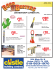### Remotec Andros Titus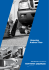### How to use Encyclopedia How to use Encyclopedia Britannica Online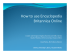### CodeLite Manual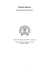### CareerScope, GCIS, and GACollege411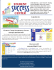### SmarAct will design a mechanical NSOM probe holder.